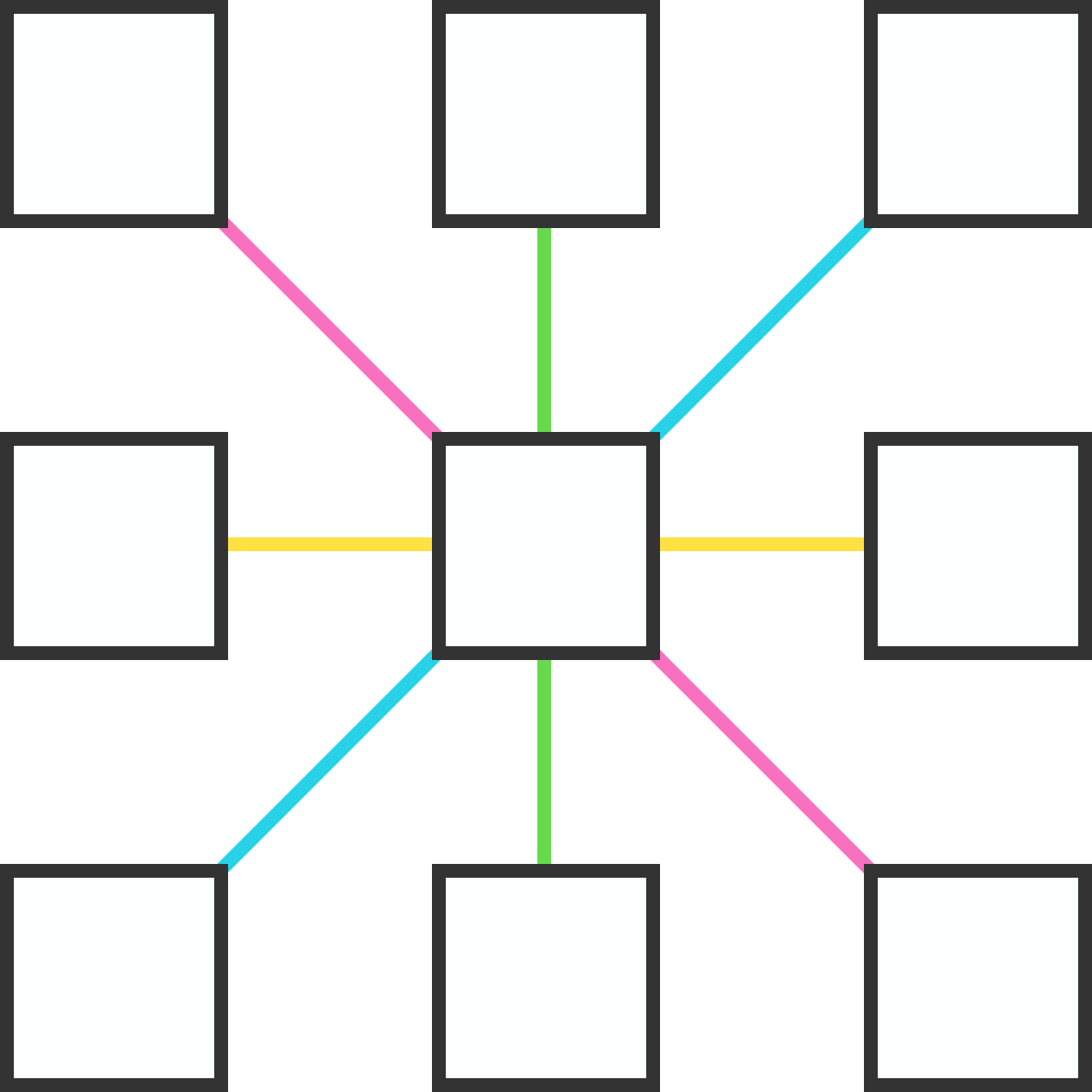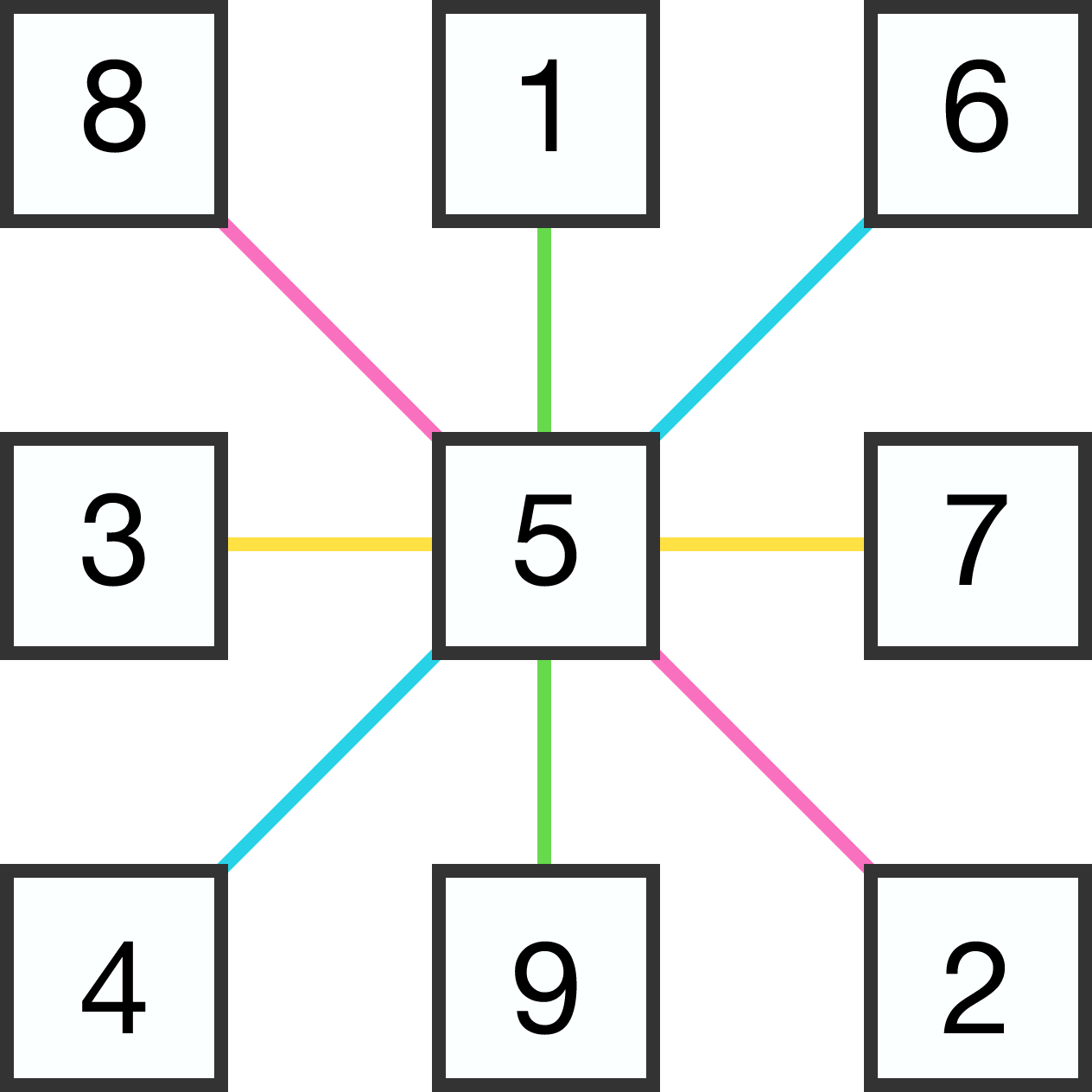# Almost A Magic Square

Algebra Level 2Place distinct digits from 1 to 9 in the boxes so that the sums of 3 digits along any of the 4 colored lines are all equal.

What is the maximum possible sum that can be achieved?

For example, using the values in the magic square gives us a sum of 15 along each colored line. Can we do better?×